# Probability of Independent Events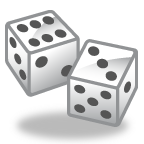Independent Events: While playing a board game, you must
toss two dice to determine how far you move on the game board.
The number that you roll on the second die is not affected
by the number that was rolled on the first die. Because the
one outcome does not affect the other, this event is called independent.

Examples:

1.  What is the probability of rolling a 5 on both of the die.

Step 1: Determine the probability of rolling a 5.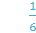Step 2: Multiply the probability of getting a 5 on each die.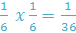This is the probability of getting a 5 on both.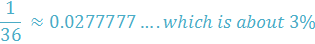It is not very likely that you will roll two fives!

2.  What is the probability of rolling a number less than three on the first die and a number greater than or equal to three on the second die?

Step 1: Determine the probability of each event.

A number less than 3: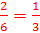A number equal to or greater than 3: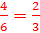Step 2: Multiply the probabilities together.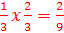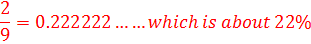There is about a 22% chance of these two events occurring together.

Let's check out coins.

3.  What is the probability of tossing a coin

twice and having both tosses land on heads.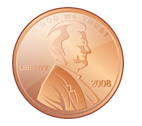What is the probability of a coin landing on heads?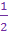The first coin toss does not affect the outcome of the second. So these are independent events.

We will multiply the probability of landing on heads for each coin.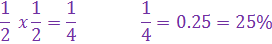There is a 25% chance of landing on heads twice.

4.  What is the probability of tossing a coin three times and having all three land on heads?

We already know that the probability of landing on heads is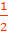.

There are three tosses. So we must multiply three probabilities.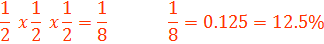There is a 12.5% chance of all three tosses landing on heads.

Now let's put both dice and coins together.

5.  What is the probability of rolling a number

less than 4 and tossing a coin that lands on tails?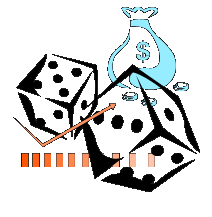The outcome on the die does not affect the outcome of the coin. Therefore, these two events are independent.

Determine the probability of each occurring and then multiply.

Probability of getting a number less than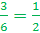Probability of getting tails =Probability of both occurring: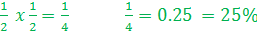Let's Review:
When the outcome of one event does not change or affect the outcome of another event, we call the two events independent. To determine the probability of both, or all, events occurring, you must determine the probability of each separate event and then multiply the events together. You can then convert the probability into a decimal or percent as needed.